# Wald tests via statsmodels (python)

The other day on crossvalidated a question came up about interpreting treatment effect differences across different crime types. This comes up all the time in criminology research, especially interventions intended to reduce crime.

Often times interventions are general and may be expected to reduce multiple crime types, e.g. hot spots policing may reduce both violent crimes and property crimes. But we do not know for sure – so it makes sense to fit models to check if that is the case.

For crimes that are more/less prevalent, this is a case in which fitting Poisson/Negative Binomial models makes alot of sense, since the treatment effect is in terms of rate modifiers. The crossvalidated post shows an example in R. In the past I have shown how to stack models and do these tests in Stata, or use seemingly unrelated regression in Stata for generalized linear models. Here I will show an example in python using data from my dissertation on stacking models and doing Wald tests.

The above link to github has the CSV file and metadata to follow along. Here I just do some upfront data prep. The data are crime counts at intersections/street segments in DC, across several different crime types and various aspects of the built environment.

``````# python code to stack models and estimate wald tests
import pandas as pd
import numpy as np
import statsmodels.formula.api as smf
import patsy
import itertools

# Use dissertation data for multiple crimes
#https://github.com/apwheele/ResearchDesign/tree/master/Week02_PresentingResearch

# only keep a few independent variables to make it simpler
crime = ['OffN1','OffN3','OffN5','OffN7','OffN8','OffN9'] #dropping very low crime counts
x = ['CFS1','CFS2'] #311 calls for service
data = data[crime + x].copy()
data.reset_index(inplace=True)

# Stack the data into long format, so each crime is a new row
data_long = pd.wide_to_long(data, 'OffN',i='MarID',j='OffCat').reset_index()``````

And here you can see what the data looks like before (wide) and after (long). I am only fitting one covariate here (detritus 311 calls for service, see my paper), which is a measure of disorder in an area.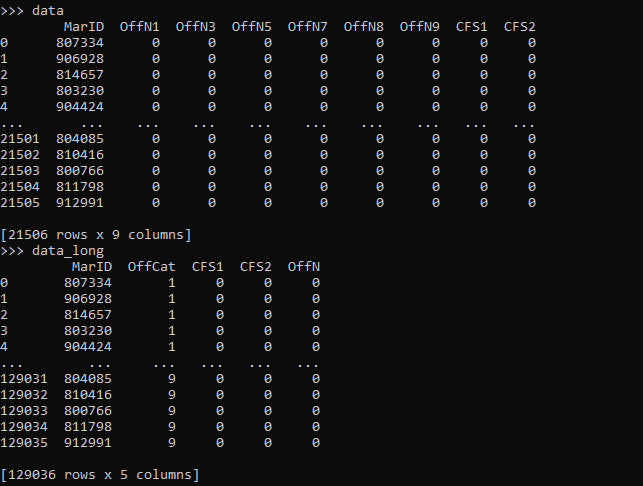For reference the offense categories are below, and I drop homicide/arson/sex abuse due to very low counts.

``````'''
Offense types in the data
OffN2   Arson #drop
OffN3   Burglary
OffN4   Homicide #drop
OffN5   Robbery
OffN6   Sex Abuse #drop
OffN7   Stolen Auto
OffN8   Theft
OffN9   Theft from Auto
'''``````

Now we can fit our stacked negative binomial model. I am going right to negative binomial, since I know this data is overdispersed and Poisson is not a good fit. I account for the clustering induced by stacking the equations, although with such a large sample it should not be a big deal.

``````# Fit a model with clustered standard errors
covp = {'groups': data_long['MarID'],'df_correction':True}
nb_mod = smf.negativebinomial('OffN ~ C(OffCat) + CFS1:C(OffCat) - 1',data_long).fit(cov_type='cluster',cov_kwds=covp)
print(nb_mod.summary())``````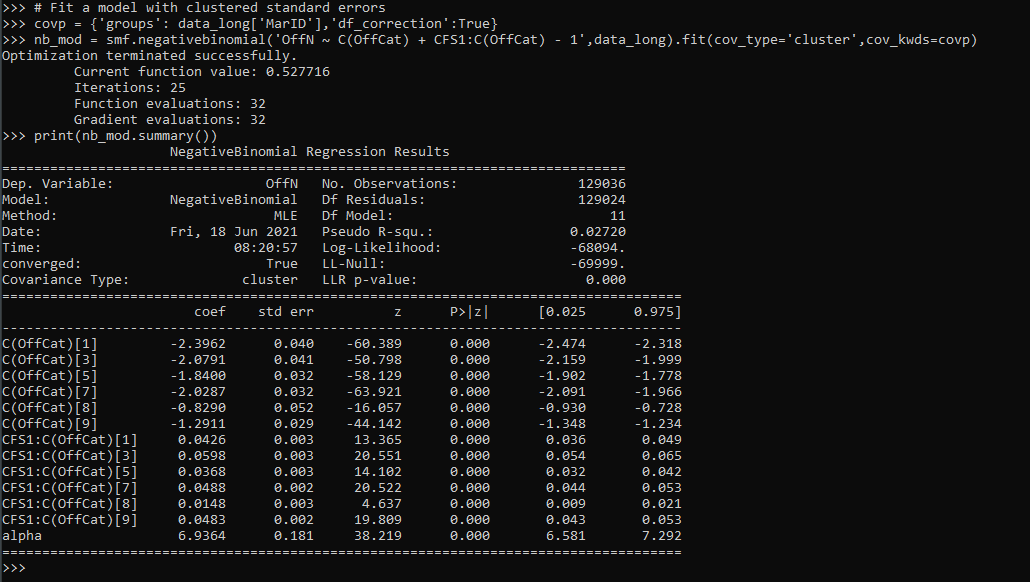So this is close to the same if you fit a separate regression for each crime type. You get an intercept for each crime type (the `C(OffCat[?])` coefficients), as well as a varying treatment effect for calls for service across each crime type, e.g. `CFS1:C(OffCat)` is the effect of 311 calls to increase Assaults, `CFS1:C(OffCat)` is to increase Burglaries, etc.

One limitation of this approach is that alpha here is constrained to be equal across each crime type. (Stata can get around this, either with the stacked equation fitting an equation for alpha based on the offense categories, or using the suest command.) But it is partly sweating the small stuff, the mean equation is the same. (So it may also make sense to not worry about clustering and fit a robust covariance estimate to the Poisson equation.)

Now onto the hypothesis tests. Besides seeing whether any individual coefficient equals 0, we may also have two additional tests. One is whether the treatment effect is equal across the different crime types. Here is how you do that in python for this example:

``````# Conduct a Wald test for equality of multiple coefficients
x_vars = nb_mod.summary2().tables.index
wald_str = ' = '.join(list(x_vars[6:-1]))
print(wald_str)
wald_test = nb_mod.wald_test(wald_str) # joint test
print(wald_test)``````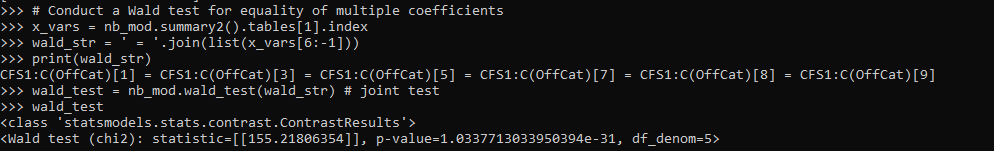Given the large sample size, even though all of the coefficients for garbage 311 calls are very similar (mostly around 0.3~0.5), this joint test says they do not all equal each other.

So the second hypothesis people are typically interested in is whether the coefficients all equal zero, a joint test. Here is how you do that in python statsmodels. They have a convenient function `.wald_test_terms()` to do just this, but I also show how to construct the same string and use `.wald_test()`.

``````# Or can do test all join equal 0
nb_mod.wald_test_terms()
# To replicate what wald_test_terms is doing yourself
all_zero = [x + '= 0' for x in x_vars[6:-1]]
nb_mod.wald_test(','.join(all_zero))``````

So we have established that when testing the equality between the coefficients, we reject the null. But this does not tell us which contrasts are themselves different and the magnitude of those coefficient differences. We can use .`t_test()` for that. (Which is the same as a Wald test, just looking at particular contrasts one by one.)

``````# Pairwise contrasts of coefficients
# To get the actual difference in coefficients
wald_li = []
for a,b in itertools.combinations(x_vars[6:-1],2):
wald_li.append(a + ' - ' + b + ' = 0')

wald_dif = ' , '.join(wald_li)

dif = nb_mod.t_test(wald_dif)
print(dif)

# c's correspond to the wald_li list
res_contrast = dif.summary_frame()
res_contrast['Test'] = wald_li
res_contrast.set_index('Test', inplace=True)
print(res_contrast)``````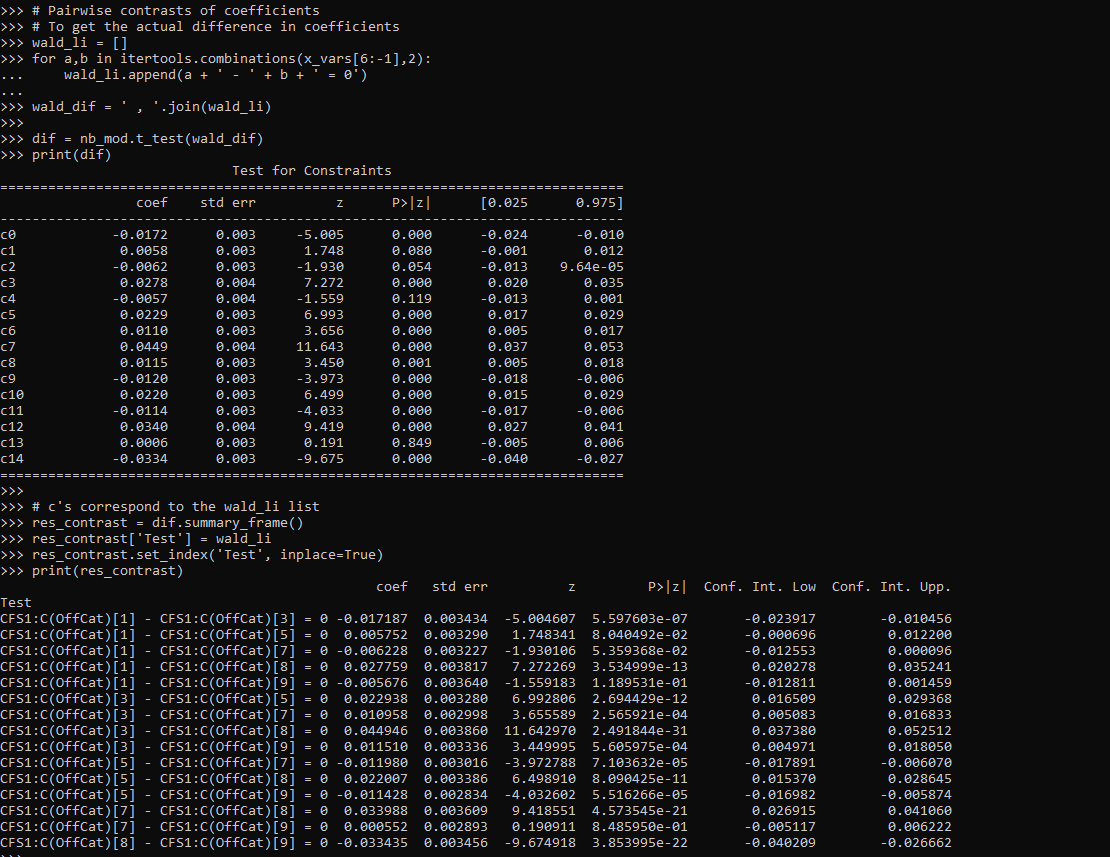You can see the original t-test table does not return a nice set of strings illustrating the actual test. So I show here how they correspond to a particular hypothesis. I actually wrote a function to give nice labels given an input test string (what you would submit to either `.t_test()` or `.wald_test()`).

``````# Nicer function to print out the actual tests it interprets as
# ends up being 1 - 3, 3 - 5, etc.
def nice_lab_tests(test_str,mod):
# Getting exogenous variables
x_vars = mod.summary2().tables.index
# Patsy getting design matrix and constraint from string
di = patsy.DesignInfo(x_vars)
const_mat = di.linear_constraint(test_str)
r_mat = const_mat.coefs
c_mat = list(const_mat.constants)
# Loop over the tests, get non-zero indices
# Build the interpreted tests
lab = []
for i,e in enumerate(c_mat):
lm = r_mat[i,:] #single row of R matrix
nz = np.nonzero(lm).tolist() #only need non-zero
c_vals = lm[nz].tolist()
v_labs = x_vars[nz].tolist()
fin_str = ''
in_val = 0
for c,v in zip(c_vals,v_labs):
# 1 and -1 drop values and only use +/-
if c == 1:
if in_val == 0:
fin_str += v
else:
fin_str += ' + ' + v
elif c == -1:
if in_val == 0:
fin_str += '-' + v
else:
fin_str += ' - ' + v
else:
if in_val == 0:
fin_str += str(c) + '*' + v
else:
if c > 0:
sg = ' + '
else:
sg = ' - '
fin_str += sg + str(np.abs(c)) + '*' + v
in_val += 1
fin_str += ' = ' + str(e) #set equality at end
lab.append(fin_str)
return lab``````

So if we look at our original `wald_str`, this converts the equality tests into a series of difference tests against zero.

``````# Wald string for equality across coefficients
# from earlier
lab_tests = nice_lab_tests(wald_str,nb_mod)
print(lab_tests)``````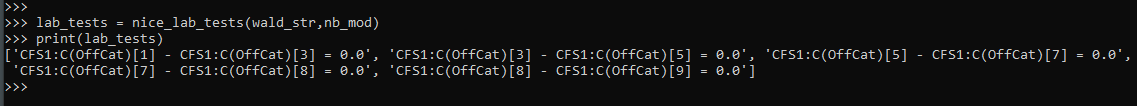And this function should work for other inputs, here is another example:

``````# Additional test to show how nice_lab_tests function works
str2 = 'CFS1:C(OffCat) = 3, CFS1:C(OffCat) = CFS1:C(OffCat)'
nice_lab_tests(str2,nb_mod)``````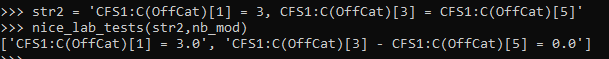Next up on the agenda is a need to figure out `.get_margeff()` a bit better for these statsmodels (or perhaps write my own closer to Stata’s implementation).

Previous Post